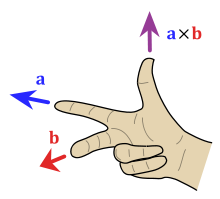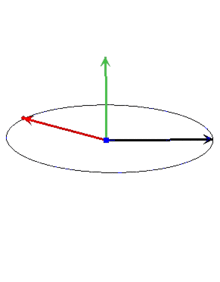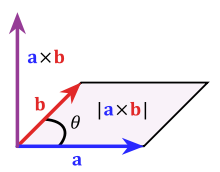# 向量点乘与叉乘

在几乎所有的几何问题中，向量（有时也称矢量）是一个基本点。向量的定义包含方向和一个数（长度）。在二维空间中，一个向量可以用一对x和y来表示。例如由点（1,3）到（5,1的向量可以用(4,-2）来表示。这里大家要特别注意，我这样说并不代表向量定义了起点和终点。向量仅仅定义方向和长度。

向量也支持各种数学运算。最简单的就是加法。我们可以对两个向量相加，得到的仍然是一个向量。我们有：
V1（x1, y1）+V2（x2, y2）=V3(x1+x2, y1+y2)
下图表示了四个向量相加。注意就像普通的加法一样,相加的次序对结果没有影响（满足交换律），减法也是一样的。

如果说加法是凭直觉就可以知道的，另外还有一些运算就不是那么明显的，比如点乘和叉乘。
点乘比较简单，是相应元素的乘积的和：
V1( x1, y1)   V2(x2, y2) = x1*x2 + y1*y2
注意结果不是一个向量，而是一个标量（Scalar）。点乘有什么用呢，我们有：
A   B = |A||B|Cos(θ)
θ是向量A和向量B见的夹角。这里|A|我们称为向量A的模(norm)，也就是A的长度， 在二维空间中就是|A| = sqrt(x2+y2)。这样我们就和容易计算两条线的夹角    Cos(θ) = AB /(|A||B|)

当然你知道要用一下反余弦函数acos()啦。（回忆一下cos(90)=0 和cos(0) = 1还是有好处的，希望你没有忘记。）这可以告诉我们如果点乘的结果，简称点积，为0的话就表示这两个向量垂直。当两向量平行时，点积有最大值
另外，点乘运算不仅限于2维空间，他可以推广到任意维空间。（译注：不少人对量子力学中的高维空间无法理解，其实如果你不要试图在视觉上想象高维空间，而仅仅把它看成三维空间在数学上的推广，那么就好理解了）

相对于点乘，叉乘可能更有用吧。2维空间中的叉乘是：
V1(x1, y1) X V2(x2, y2) = x1y2 – y1x2
看起来像个标量，事实上叉乘的结果是个向量，方向在z轴上。上述结果是它的模。在二维空间里，让我们暂时忽略它的方向，将结果看成一个向量，那么这个结果类似于上述的点积，我们有：
A x B = |A||B|Sin(θ)

然而角度 θ和上面点乘的角度有一点点不同，他是有正负的，是指从A到B的角度。因此 ，向量的外积不遵守乘法交换率，因为向量a×向量b=-向量b×向量a在物理学中，已知力与力臂求外积，就是向量的外积，即叉乘。

向量c的方向与a,b所在的平面垂直，且方向要用“右手法则”判断。判断方法如下：

1.右手手掌张开，四指并拢，大拇指垂直于四指指向的方向；
2.伸出右手，四指弯曲，四指与A旋转到B方向一致，那么大拇指指向为C向量的方向。另外还有一个有用的特征那就是叉积的绝对值就是A和B为两边说形成的平行四边形的面积。也就是AB所包围三角形面积的两倍。在计算面积时，我们要经常用到叉积。（译注：三维及以上的叉乘参看维基：http://en.wikipedia.org/wiki/Cross_product）

-线距离
找出一个点和一条线间的距离是经常遇见的几何问题之一。假设给出三个点，A，B和C，你想找出点C到点A、B定出的直线间距离。第一步是找出A到B的向量AB和A到C的向量AC，现在我们用该两向量的叉积除以|AB|，这就是我们要找的的距离了（下图中的红线）。
d = (AB x AC)/|AB|

如果你有基础的高中几何知识，你就知道原因了。上一节我们知道(AB X AC)/2是三角形ABC的面积，这个三角形的底是|AB|，高就是C到AB的距离。有时叉积得到的是一个负值，这种情况下距离就是上述结果的绝对值。
当我们要找点到线段的距离时，情况变得稍稍复杂一些。这时线段与点的最短距离可能是点到线段的某一端点，而不是点到直线的垂线。例如上图中点C到线段AB的最短距离应该是线段BC。我们有集中不同的方法来判断这种特殊情况。第一种情况是计算点积AB B来判定两线段间夹角。如果点积大于等于零，那么表示AB到BC是在-90到90度间，也就是说C到AB的垂线在AB外，那么AB上到C距离最近的点就是B。同样，如果BAAC大于等于零，那么点A就是距离C最近的点。如果两者均小于零，那么距离最近的点就在线段AB中的莫一点。

//Compute the dot product AB   BC
int dot(int[] A, int[] B, int[] C){
AB = new int;
BC = new int;
AB = B-A;
AB = B-A;
BC = C-B;
BC = C-B;
int dot = AB * BC + AB * BC;
return dot;
}
//Compute the cross product AB x AC
int cross(int[] A, int[] B, int[] C){
AB = new int;
AC = new int;
AB = B-A;
AB = B-A;
AC = C-A;
AC = C-A;
int cross = AB * AC - AB * AC;
return cross;
}
//Compute the distance from A to B
double distance(int[] A, int[] B){
int d1 = A - B;
int d2 = A - B;
return sqrt(d1*d1+d2*d2);
}
//Compute the distance from AB to C
//if isSegment is true, AB is a segment, not a line.
double linePointDist(int[] A, int[] B, int[] C, boolean isSegment){
double dist = cross(A,B,C) / distance(A,B);
if(isSegment){
int dot1 = dot(A,B,C);
if(dot1 > 0)return distance(B,C);
int dot2 = dot(B,A,C);
if(dot2 > 0)return distance(A,C);
}
return abs(dist);
}

上面的代码看起来似乎是很繁琐。不过我们可以看看在C++和C#中，采用了运算符重载的类point，用‘*’代表点乘，用'^'代表叉乘（当然'+''-'还是你所希望的），那么看起来就简单些，代码如下：

//Compute the distance from AB to C
//if isSegment is true, AB is a segment, not a line.
double linePointDist(point A, point B, point C, bool isSegment){
double dist = ((B-A)^(C-A)) / sqrt((B-A)*(B-A));
if(isSegment){
int dot1 = (C-B)*(B-A);
if(dot1 > 0)return sqrt((B-C)*(B-C));
int dot2 = (C-A)*(A-B);
if(dot2 > 0)return sqrt((A-C)*(A-C));
}
return abs(dist);
}
posted on 2017-09-12 10:18  莫水千流  阅读(6567)  评论(0编辑  收藏  举报